基于DCT的数字水印算法

三、数字水印的定义和基本特点

(1) 不可见性。在宿主数字媒体中嵌入一定数量的附加信息后，不能引起明显的将质现象，隐藏的数据不易觉察，即无法人为的看见或听见。

(2) 稳健性。数字水印必须对施加于宿主媒体的变化或操作具有一定的免疫力，不能因为某种变换操作导致水印信息的丢失，即水印被迫坏，从而失去商用价值。常用的变换操作有：信道噪声、滤波、有损压缩、重采样等。

(3) 安全性。数字水印应该能够抵抗各种蓄意的攻击，同时应很难被他人复制和伪造。

(4) 有效性。水印提取算法应高效，提取出的水印应能唯一标识版权所有者。

(5)抗窜改性。 与抗毁坏的鲁棒性不同,抗窜改性是指水印一旦嵌入到载体中,攻击者就很难改变或伪造。鲁棒性要求高的应用，通常也需要很强的抗窜改性，在版权保护中,要达到好的抗窜改性是比较困难的。

五、各种数字水印算法

1. 空间域算法

(1) 最低有效位方法(LSB)

(2) Patchwork方法及纹理块映射编码方法

2.变换域算法

3.NEC算法

4.其他一些水印算法

六、MATLAB参考程序

1  size=512; N=32;K=8; D=zeros(size);E=0.01;
3  subplot(2,2,1);
4  imshow(I);
5  title('原始图像');
6  I=double(I)/512;
%读入水银
8  subplot(2,2,2);
9  imshow(J);
10 title('水印图像');
11 %对水印缩放
12 J=double(imresize(J,[64,64]));
13 subplot(2,2,3);
41 imshow(J);
15 title('缩放水印图像');
16 %嵌入水印
17 for p=1:size/K
18     for q=1:size/K
19         x=(p-1)*K+1; y=(q-1)*K+1;
20         I_dct=I(x:x+K-1,y:y+K-1);
21       I_dct1=dct2(I_dct);
22       if J(p,q)==0
23           alfa=-1;
24       else
25          alfa=1;
26       end
27      I_dct2=I_dct1+alfa*E;
28       I_dct=idct2(I_dct2);
29       D(x:x+K-1,y:y+K-1)=I_dct;
30   end
31 end
32 subplot(1,3,3);
33 imshow(D,[]);
34 Title('含水印的图象');
35 %%%%%%对加入水印的图像进行各种攻击
36 %%%低通滤波攻击
37 %hh=fspecial('gaussian',3,0.2);
38 %hh=fspecial('gaussian',3,0.35);
39 %hh=fspecial('gaussian',3,0.4);
40 QQ=filter2(hh,D);
41%%%旋转攻击
42 R=imrotate(D,10,'bilinear','crop');
43 %R=imrotate(D,20,'bilinear','crop');
44 %R=imrotate(D,45,'bilinear','crop');
45 %%%%%剪切攻击
46 %D(1:32,1:32)=0;   Q2=D;
47 %D(1:64,1:64)=0;   Q2=D;
48 %D(1:128,1:128)=0;  Q2=D;
49 % 提取水印
50 for  p=1:size/K
51 for  q=1:size/K
52       x=(p-1)*K+1;
53       y=(q-1)*K+1;
54       I1=I(x:x+K-1,y:y+K-1);
55       I2=QQ(x:x+K-1,y:y+K-1);
56       I_dct1=dct2(I1);
57       I_dct2=dct2(I2);
58       if  I_dct2>I_dct1
59         W(p,q)=1;
60       else
61          W(p,q)=0;
62       end
63  end
64 end
65 figure,subplot(1,2,1);imshow(R,[]);title('低通滤波攻击');
66 subplot(1,2,2);imshow(W,[]);title('图像中提取的水印');
67  %%%%%%前后水印相似比较
68 J=double(J);W=double(W);
69 sumJ=0;sumW=0;
70 for  j=1:N
71 for  i=1:N
72     sumJ=sumJ+J(j,i)*W(j,i);
73     sumW=sumW+W(j,i)*W(j,i);
74  end
75 end
76 CH=(sumW-sumJ)/sumJ

七、运行结果比较

1.1---34行程序运行结果如下：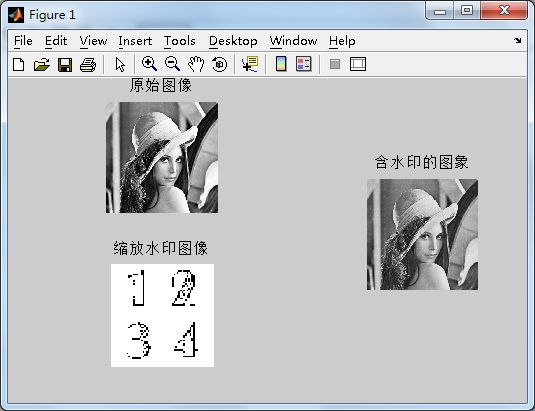2.不同程度的低通滤波攻击图像及提出的水印信息：

(1)hh=fspecial('gaussian',3,0.2)攻击与提取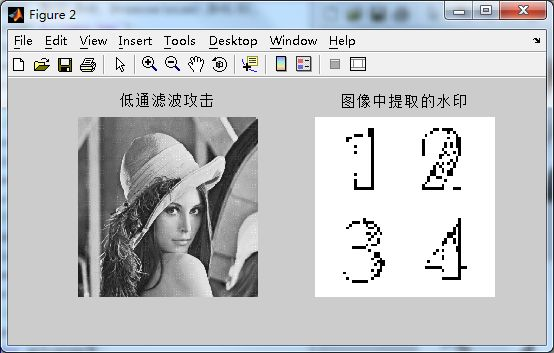(2)hh=fspecial('gaussian',3,0.35) 攻击与提取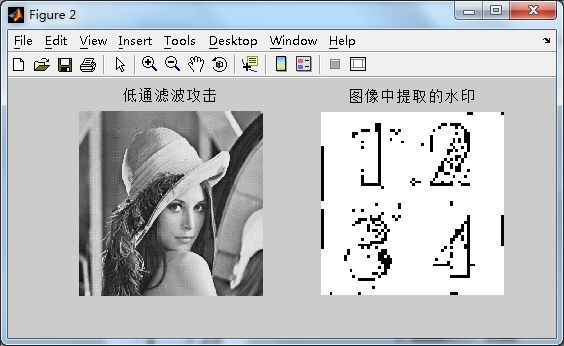(3)hh=fspecial('gaussian',3,0.4)攻击与提取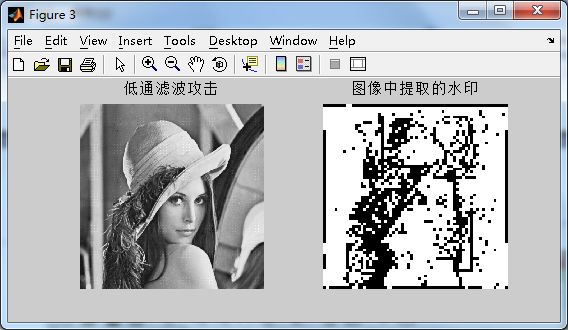3.不同程度的旋转攻击图像及提出的水印信息：

(1)R1=imrotate(D,10,'bilinear','crop')的攻击与提取：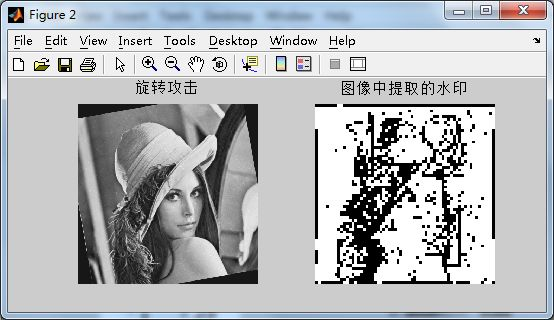(2) R2=imrotate(D,20,'bilinear','crop') 的攻击与提取：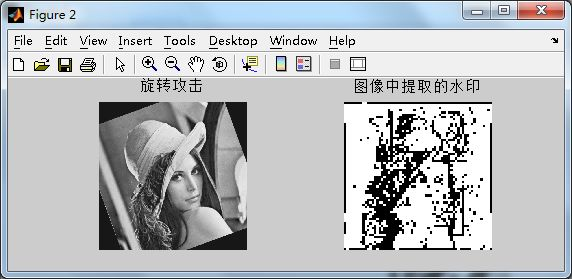(3) R3=imrotate(D,45,'bilinear','crop') 的攻击与提取：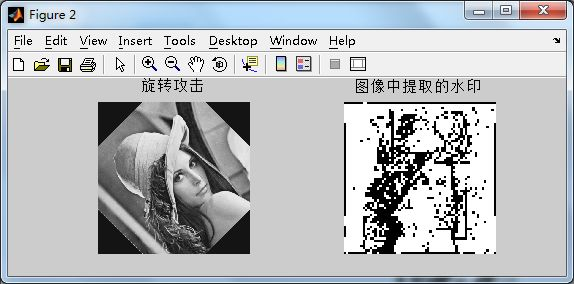4. 不同程度的剪切攻击图像及提出的水印信息：

(1)D(1:32,1:32)=0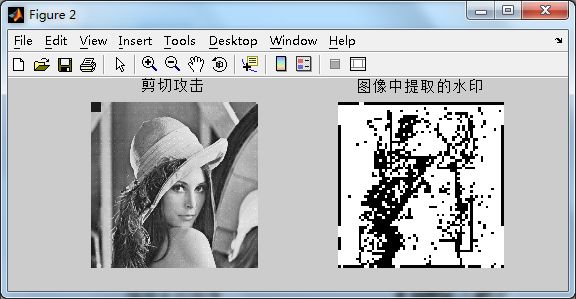(2)D(1:64,1:64)=0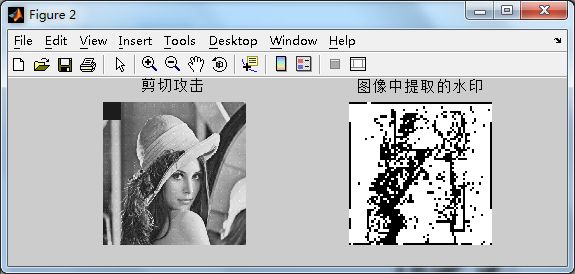(3)D(1:128,1:128)=0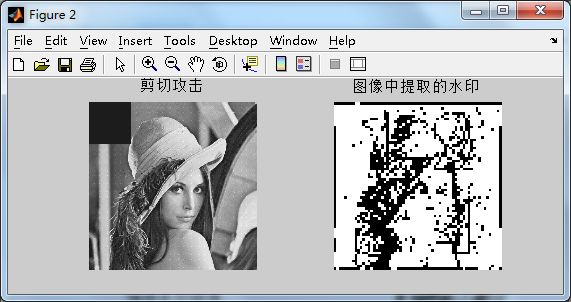5. 不同嵌入深度的图像的比较：

E=0.01 E=0.1 E=1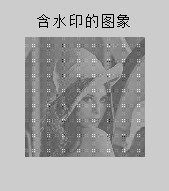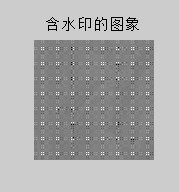八、提取水印与原始水印的相似程度比较

 各种攻击 相似程度 旋转攻击 旋转10度 0.9955 低通滤波攻击hh=fspecial('gaussian',3,0.2) 0.9955 剪切攻击 D(1:32,1:32)=0 0.9955

11-21
08-1017611-217397
09-013181
05-153万+
03-1666
06-26565
12-01
08-28758
04-283786
12-125169
10-263万+
12-241233
06-04
03-157938
04-22yl624624

¥2 ¥4 ¥6 ¥10 ¥20余额支付 (余额：-- )扫码支付获取中扫码支付点击重新获取扫码支付1.余额是钱包充值的虚拟货币，按照1:1的比例进行支付金额的抵扣。
2.余额无法直接购买下载，可以购买VIP、C币套餐、付费专栏及课程。余额充值